# scipy.spatial.distance.hamming¶

scipy.spatial.distance.hamming(u, v)[source]

Computes the Hamming distance between two 1-D arrays.

The Hamming distance between 1-D arrays u and v, is simply the proportion of disagreeing components in u and v. If u and v are boolean vectors, the Hamming distance is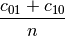where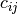is the number of occurrences of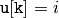and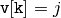for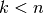.

Parameters : u : (N,) array_like Input array. v : (N,) array_like Input array. hamming : double The Hamming distance between vectors u and v.

#### Previous topic

scipy.spatial.distance.euclidean

#### Next topic

scipy.spatial.distance.jaccard